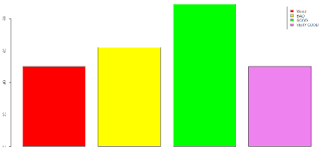# BigDataNews

A Data Science Central Community

Today I will explain you how to create a basic Movie review engine based on the tweets by people using R. The implementation of the Review Engine will be as follows:
• Clean the data
• Create a Word Cloud
• Create a data dictionary
• Score each tweet.

First step is to fetch the data from Twitter. In R, we have facility to call the twitter API using package twitter. Below are the steps for fetch the tweets using twitter package. Each tweet data contains:
• Text
• Is re-tweeted
• Re-tweet count
• Tweeted User name
• Latitude/Longitude
• Replied to, etc.

For our case we only consider Text feature of the Tweet as we are interested on the review of the movie. We can also use the other features such as Latitude/Longitude, replied to, etc. do other analysis on the tweeted data.

library(tm)Clean the data:
In the next step, we need to clean the data so that we can use it for our analysis. Cleaning of data is a very important step in Data Analysis. This step includes:
Extracting only text from Tweets:
tweets_txt = sapply(tweets,function(x) x\$getText())
tweets_cl = gsub("(RT|via)((?:\\b\\W*@\\w+)+)","",tweets)
tweets_cl = gsub("http[^[:blank:]]+", "", tweets_cl)
tweets_cl = gsub("@\\w+", "", tweets_cl)
tweets_cl = gsub("[ \t]{2,}", "", tweets_cl)
tweets_cl = gsub("^\\s+|\\s+\$", "", tweets_cl)
tweets_cl = gsub("[[:punct:]]", " ", tweets_cl)
tweets_cl = gsub("[^[:alnum:]]", " ", tweets_cl)
tweets_cl <- gsub('\\d+', '', tweets_cl)
Create a Word Cloud:
At this point let us view Word-Cloud of frequently tweeted words in the data considered for visual understanding/analyzing the data.
library(wordcloud)
wordcloud(tweets_cl)Create a data dictionary:
In this step, we create use a Dictionary of words containing positive, negative words which are downloaded from here. These 2 types of words are used as keywords for classifying the each tweet into one of the 4 categories: Very Positive, Positive, Negative and Very Negative.
Score each tweet:
In this step, we will write a function which will calculate rating of the movie. The function is given below. After calculating the scores we plot graphs showing the rating as “WORST”,”BAD”,”GOOD”,”VERYGOOD”Future steps in this project will be:
• To create a UI preferably using .NET, as I’m a dot-net developer ;)
• To Build a Movie Review Model which can classify a new tweet as and when provided?

Code:

#include required libraries
library(plyr)
library(stringr)
#get the tweets
tweets_txt = sapply(tweets[1:50],function(x) x\$getText())
#function to clean data
cleanTweets = function(tweets)
{
tweets_cl = gsub("(RT|via)((?:\\b\\W*@\\w+)+)","",tweets)
tweets_cl = gsub("http[^[:blank:]]+", "", tweets_cl)
tweets_cl = gsub("@\\w+", "", tweets_cl)
tweets_cl = gsub("[ \t]{2,}", "", tweets_cl)
tweets_cl = gsub("^\\s+|\\s+\$", "", tweets_cl)
tweets_cl = gsub("[[:punct:]]", " ", tweets_cl)
tweets_cl = gsub("[^[:alnum:]]", " ", tweets_cl)
tweets_cl <- gsub('\\d+', '', tweets_cl)
return(tweets_cl)
}
#function to calculate number of words in each category within a sentence
sentimentScore <- function(sentences, vNegTerms, negTerms, posTerms, vPosTerms){
final_scores <- matrix('', 0, 5)
scores <- laply(sentences, function(sentence, vNegTerms, negTerms, posTerms, vPosTerms){
initial_sentence <- sentence
#remove unnecessary characters and split up by word
sentence = cleanTweets(sentence)
sentence <- tolower(sentence)
wordList <- str_split(sentence, '\\s+')
words <- unlist(wordList)
#build vector with matches between sentence and each category
vPosMatches <- match(words, vPosTerms)
posMatches <- match(words, posTerms)
vNegMatches <- match(words, vNegTerms)
negMatches <- match(words, negTerms)
#sum up number of words in each category
vPosMatches <- sum(!is.na(vPosMatches))
posMatches <- sum(!is.na(posMatches))
vNegMatches <- sum(!is.na(vNegMatches))
negMatches <- sum(!is.na(negMatches))
score <- c(vNegMatches, negMatches, posMatches, vPosMatches)
newrow <- c(initial_sentence, score)
final_scores <- rbind(final_scores, newrow)
return(final_scores)
}, vNegTerms, negTerms, posTerms, vPosTerms)
return(scores)
}
names(afinn_list) <- c('word', 'score')
afinn_list\$word <- tolower(afinn_list\$word)
#categorize words as very negative to very positive and add some movie-specific words
vNegTerms <- afinn_list\$word[afinn_list\$score==-5 | afinn_list\$score==-4]
negTerms <- c(afinn_list\$word[afinn_list\$score==-3 | afinn_list\$score==-2 | afinn_list\$score==-1], "second-rate", "moronic", "third-rate", "flawed", "juvenile", "boring", "distasteful", "ordinary", "disgusting", "senseless", "static", "brutal", "confused", "disappointing", "bloody", "silly", "tired", "predictable", "stupid", "uninteresting", "trite", "uneven", "outdated", "dreadful", "bland")
posTerms <- c(afinn_list\$word[afinn_list\$score==3 | afinn_list\$score==2 | afinn_list\$score==1], "first-rate", "insightful", "clever", "charming", "comical", "charismatic", "enjoyable", "absorbing", "sensitive", "intriguing", "powerful", "pleasant", "surprising", "thought-provoking", "imaginative", "unpretentious")
vPosTerms <- c(afinn_list\$word[afinn_list\$score==5 | afinn_list\$score==4], "uproarious", "riveting", "fascinating", "dazzling", "legendary")
#Calculate score on each tweet
tweetResult <- as.data.frame(sentimentScore(tweets_txt, vNegTerms, negTerms, posTerms, vPosTerms))
tweetResult\$'2' = as.numeric(tweetResult\$'2')
tweetResult\$'3' = as.numeric(tweetResult\$'3')
tweetResult\$'4' = as.numeric(tweetResult\$'4')
tweetResult\$'5' = as.numeric(tweetResult\$'5')
counts = c(sum(tweetResult\$'2'),sum(tweetResult\$'3'),sum(tweetResult\$'4'),sum(tweetResult\$'5'))
mr = list(counts,names)
colors = c("red", "yellow", "green", "violet")
barplot(mr[], main="Movie Review", xlab="Number of votes",legend=mr[],col=colors)

Originally posted here.

Views: 16887

Comment